Visualize boxplots and histogram by attribute of univar_numeric class.

# S3 method for univar_numeric
plot(
x,
indiv = FALSE,
viz = c("hist", "boxplot"),
stand = ifelse(rep(indiv, 4), c("none", "robust", "minmax", "zscore"), c("robust",
"minmax", "zscore", "none")),
prompt = FALSE,
typographic = TRUE,
base_family = NULL,
...
)

## Arguments

x an object of class "univar_numeric", usually, a result of a call to univar_numeric(). logical. Select whether to display information of all variables in one plot when there are multiple selected numeric variables. In case of FALSE, all variable information is displayed in one plot. If TRUE, the information of the individual variables is output to the individual plots. The default is FALSE. If only one variable is selected, TRUE is applied. character. Describe what to plot visualization. "hist" draws a histogram and "boxplot" draws a boxplot. The default is "hist". character. Describe how to standardize the original data. "robust" normalizes the raw data through transformation calculated by IQR and median. "minmax" normalizes the original data using minmax transformation. "zscore" standardizes the original data using z-Score transformation. "none" does not perform data transformation. he default is "none" if indiv is TRUE, and "robust" if FALSE. logical. The default value is FALSE. If there are multiple visualizations to be output, if this argument value is TRUE, a prompt is output each time. logical. Whether to apply focuses on typographic elements to ggplot2 visualization. The default is TRUE. if TRUE provides a base theme that focuses on typographic elements using hrbrthemes package. character. The name of the base font family to use for the visualization. If not specified, the font defined in dlookr is applied. (See details) arguments to be passed to methods, such as graphical parameters (see par). However, it does not support.

## Details

The base_family is selected from "Roboto Condensed", "Liberation Sans Narrow", "NanumSquare", "Noto Sans Korean". If you want to use a different font, use it after loading the Google font with import_google_font().

univar_numeric, print.univar_numeric, summary.univar_numeric.

## Examples

# \donttest{
# Calculates the all categorical variables
all_var <- univar_numeric(heartfailure)

# Print univar_numeric class object
all_var#> $statistics #> # A tibble: 7 x 10 #> variable n na mean sd se_mean IQR skewness kurtosis median #> <chr> <int> <int> <dbl> <dbl> <dbl> <dbl> <dbl> <dbl> <dbl> #> 1 age 299 0 6.08e1 1.19e1 6.88e-1 19 0.424 -0.184 6 e1 #> 2 cpk_enzyme 299 0 5.82e2 9.70e2 5.61e+1 466. 4.46 25.1 2.5 e2 #> 3 ejection_… 299 0 3.81e1 1.18e1 6.84e-1 15 0.555 0.0414 3.8 e1 #> 4 platelets 299 0 2.63e5 9.78e4 5.66e+3 91000 1.46 6.21 2.62e5 #> 5 creatinine 299 0 1.39e0 1.03e0 5.98e-2 0.5 4.46 25.8 1.1 e0 #> 6 sodium 299 0 1.37e2 4.41e0 2.55e-1 6 -1.05 4.12 1.37e2 #> 7 time 299 0 1.30e2 7.76e1 4.49e+0 130 0.128 -1.21 1.15e2 #> # Calculates the platelets, sodium variable univar_numeric(heartfailure, platelets, sodium)#>$statistics
#> # A tibble: 2 x 10
#>   variable      n    na    mean       sd  se_mean   IQR skewness kurtosis median
#>   <chr>     <int> <int>   <dbl>    <dbl>    <dbl> <dbl>    <dbl>    <dbl>  <dbl>
#> 1 platelets   299     0 263358. 97804.   5656.    91000     1.46     6.21 262000
#> 2 sodium      299     0    137.     4.41    0.255     6    -1.05     4.12    137
#>
# Summary the all case : Return a invisible copy of an object.
stat <- summary(all_var)

# Summary by returned object
stat#> # A tibble: 7 x 8
#>   variable              mean    sd se_mean   IQR skewness kurtosis median
#>   <chr>                <dbl> <dbl>   <dbl> <dbl>    <dbl>    <dbl>  <dbl>
#> 1 age                0.0437  0.626  0.0362     1    0.424  -0.184       0
#> 2 cpk_enzyme         0.713   2.08   0.121      1    4.46   25.1         0
#> 3 ejection_fraction  0.00557 0.789  0.0456     1    0.555   0.0414      0
#> 4 platelets          0.0149  1.07   0.0622     1    1.46    6.21        0
#> 5 creatinine         0.588   2.07   0.120      1    4.46   25.8         0
#> 6 sodium            -0.0624  0.735  0.0425     1   -1.05    4.12        0
#> 7 time               0.117   0.597  0.0345     1    0.128  -1.21        0
# one plot with all variables
plot(all_var)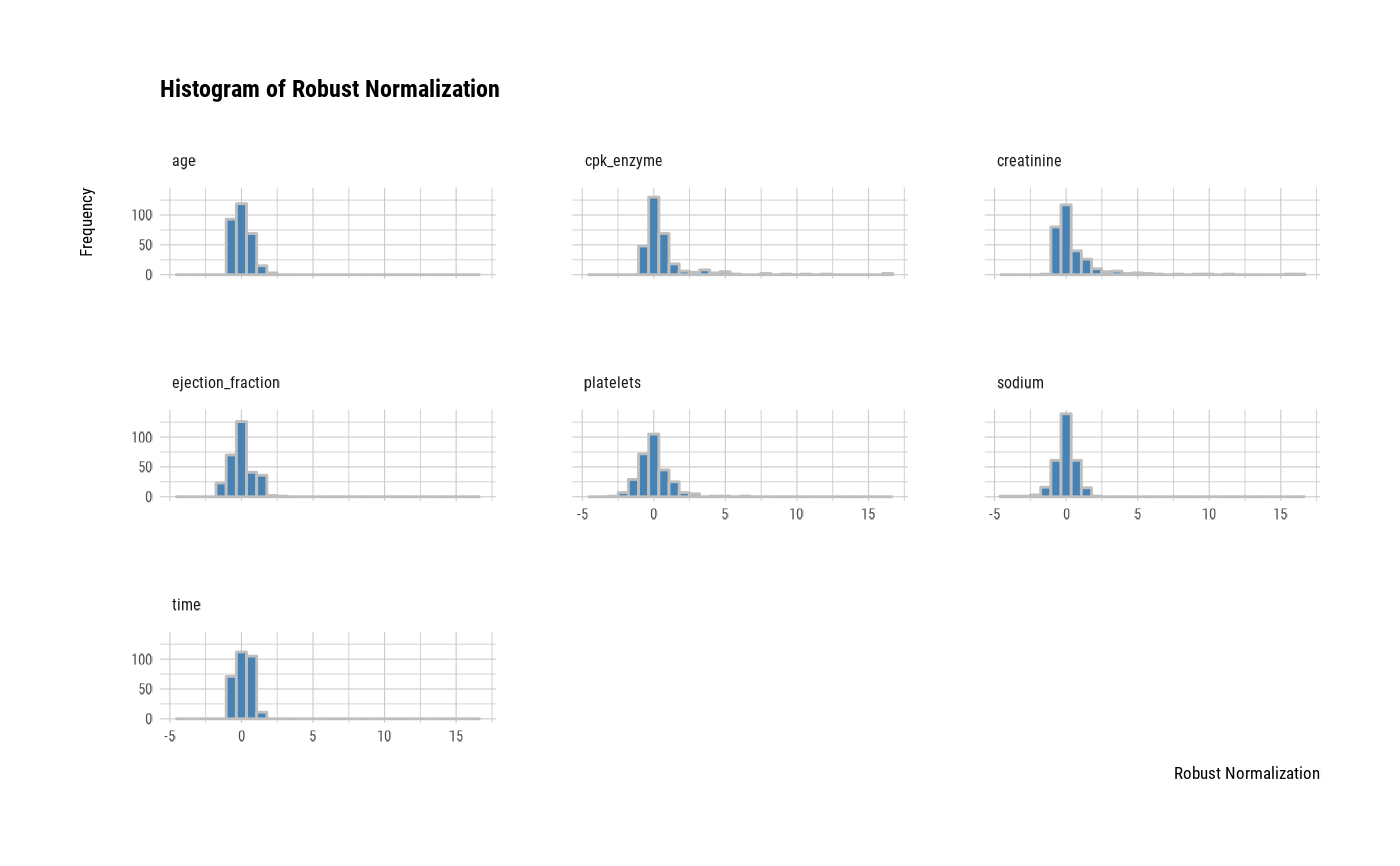# one plot with all normalized variables by Min-Max method
plot(all_var, stand = "minmax")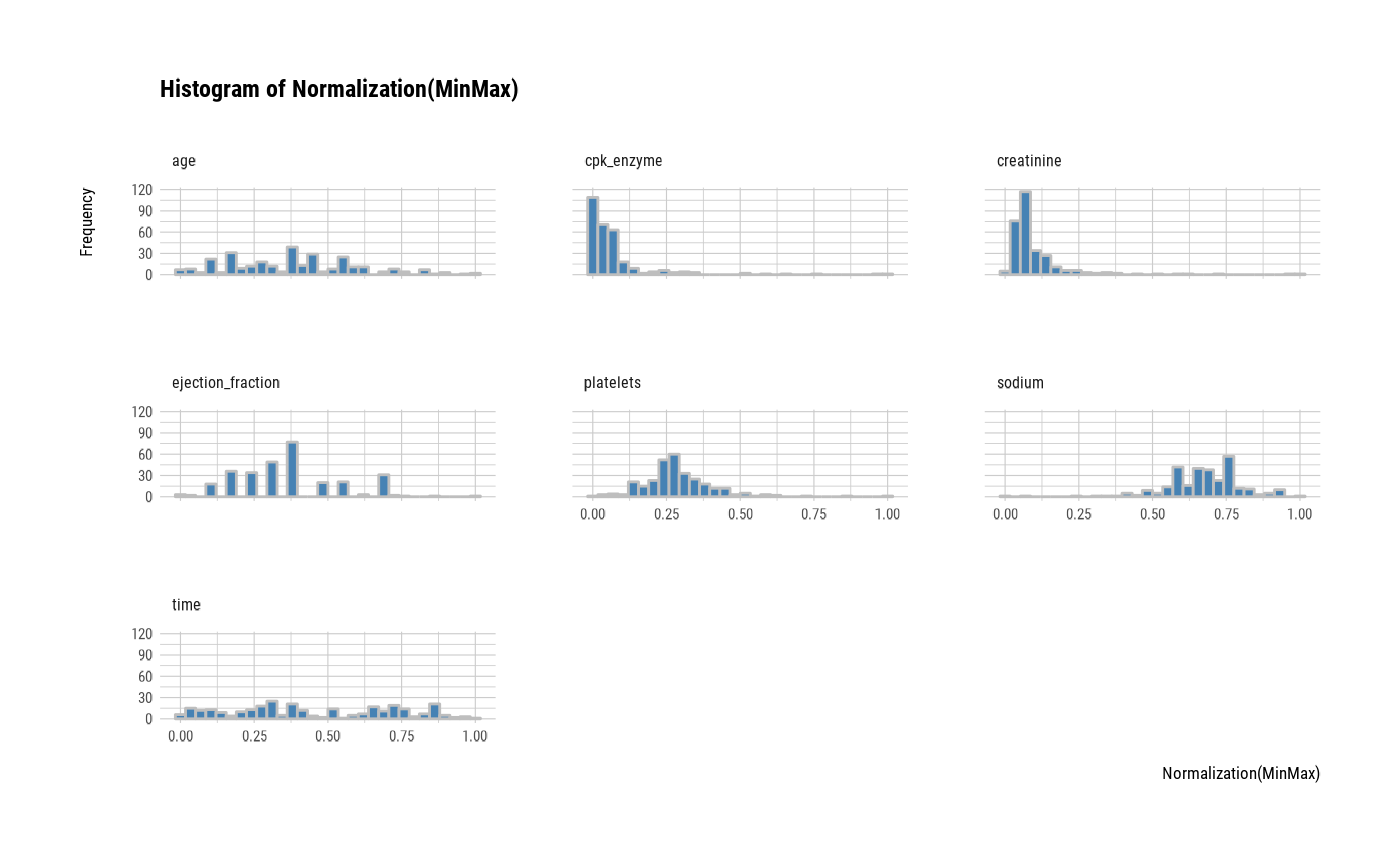# one plot with all variables
plot(all_var, stand = "none")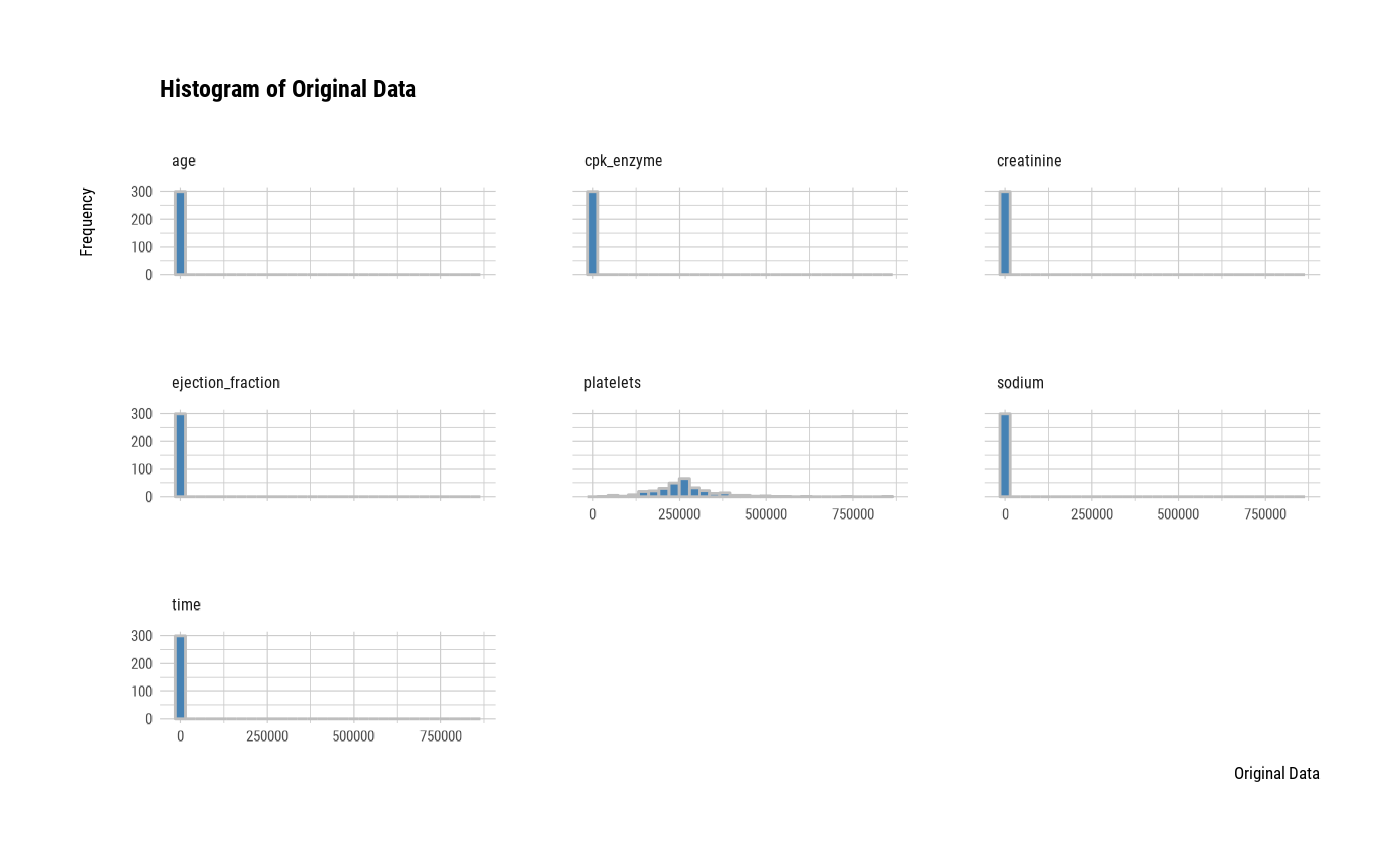# one plot with all robust standardized variables
plot(all_var, viz = "boxplot")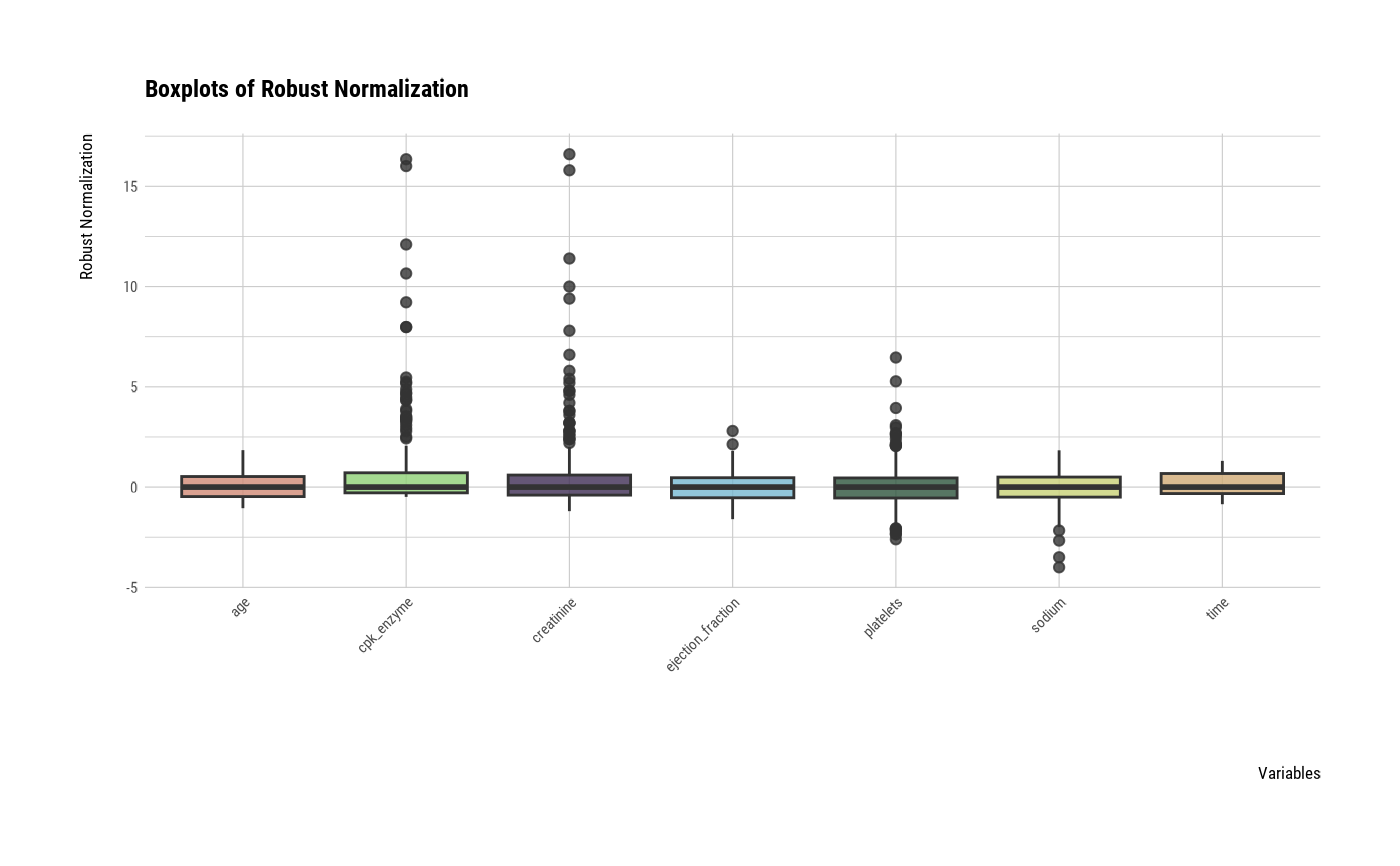# one plot with all standardized variables by Z-score method
plot(all_var, viz = "boxplot", stand = "zscore")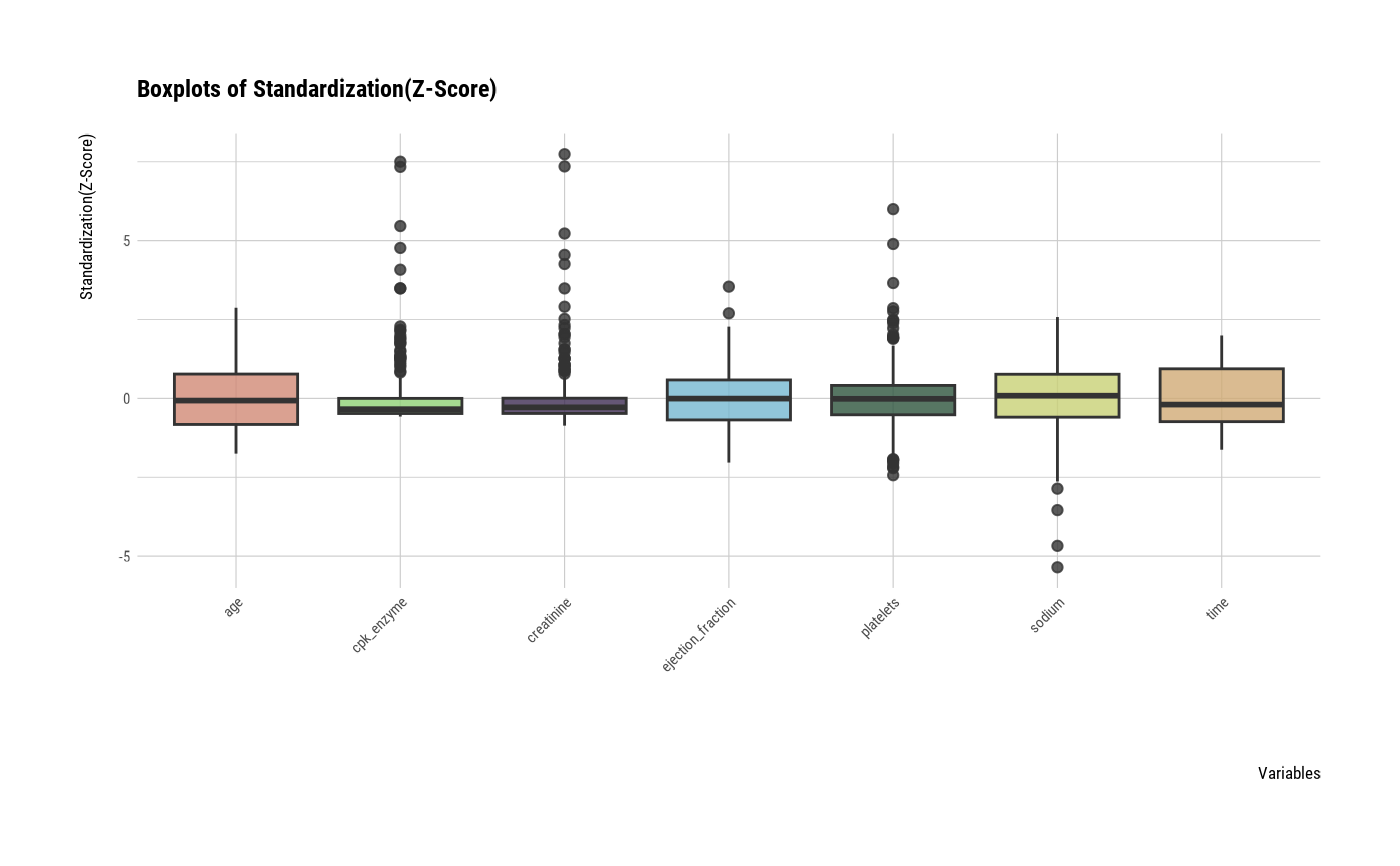# individual boxplot by variables
plot(all_var, indiv = TRUE, "boxplot")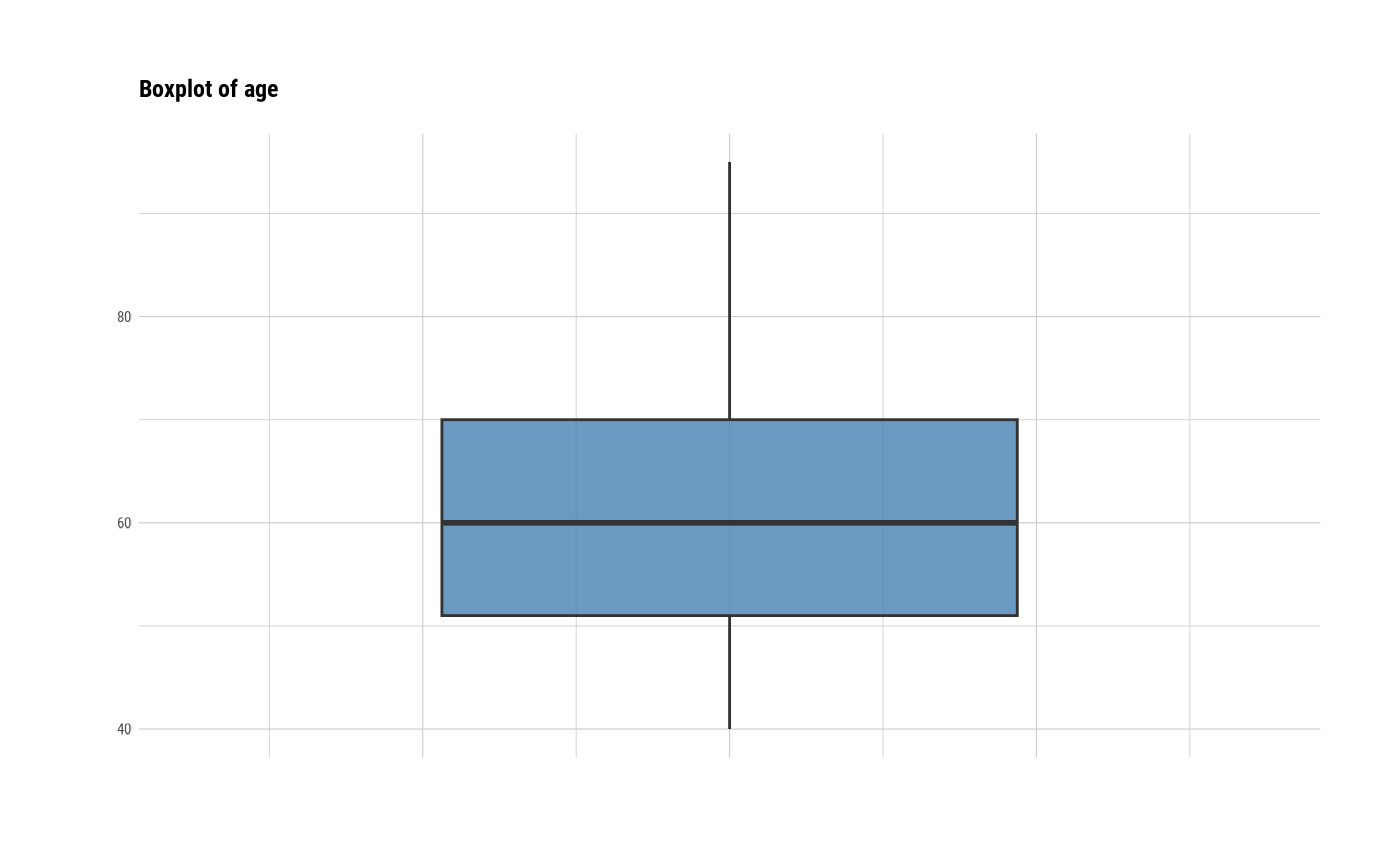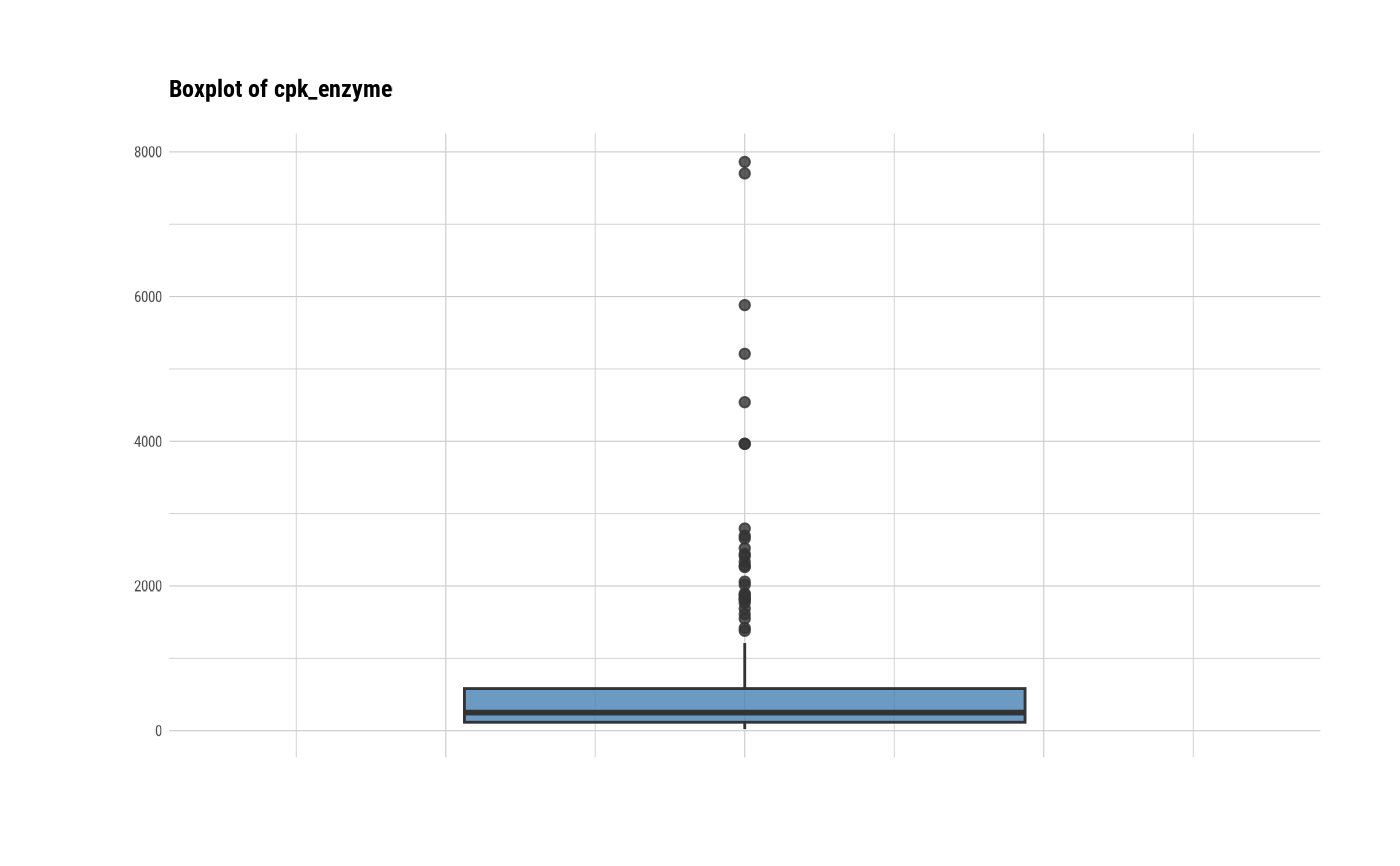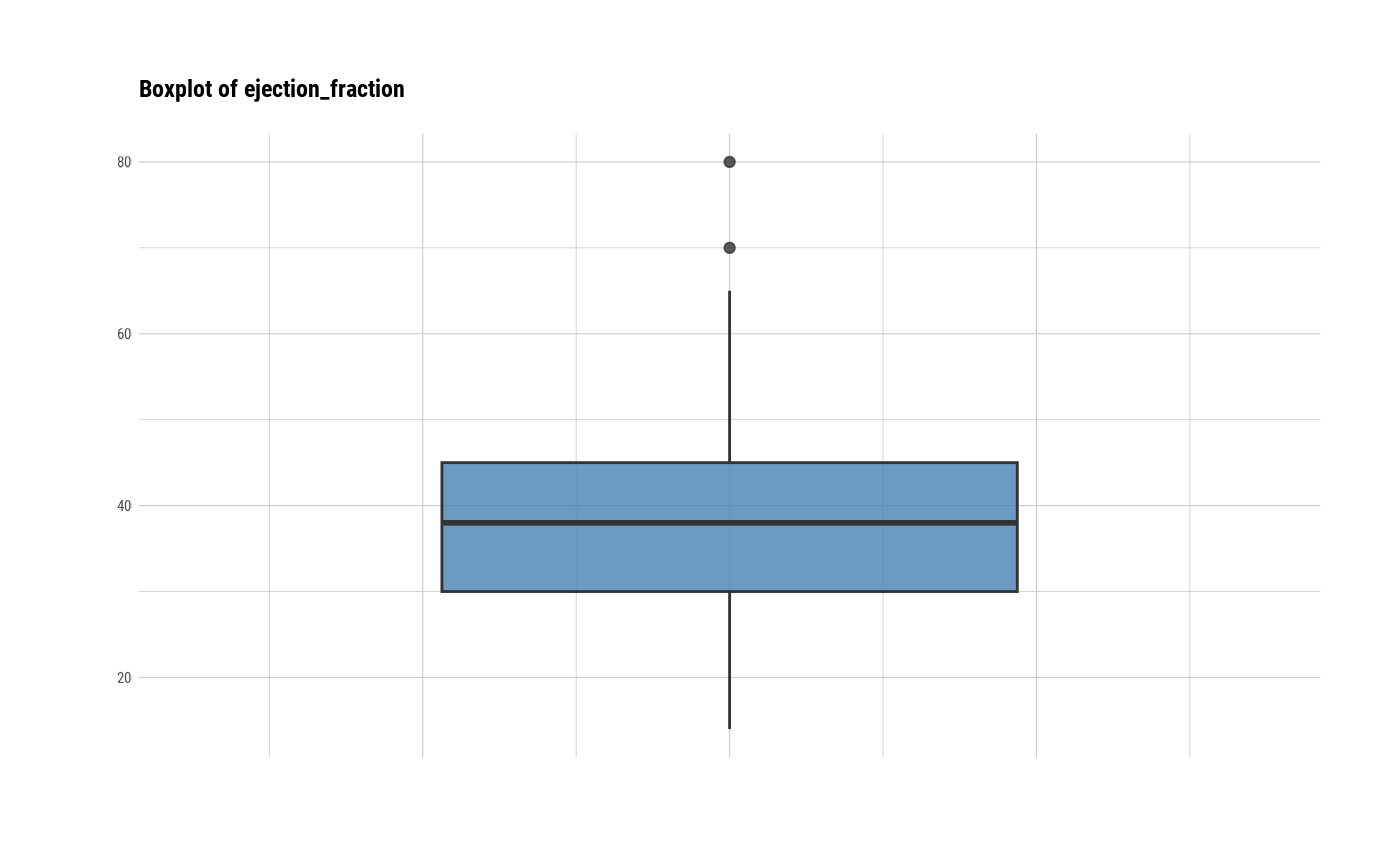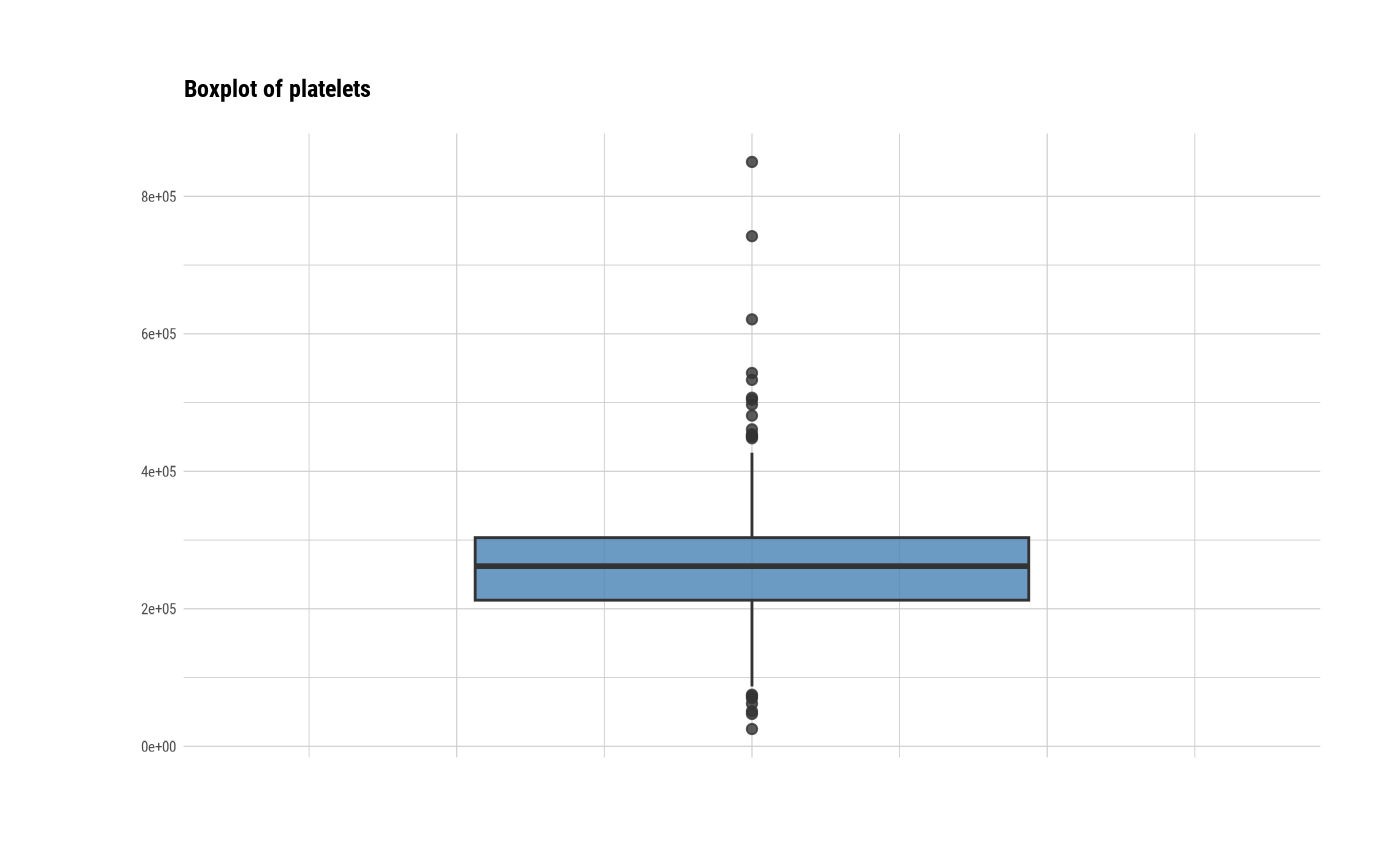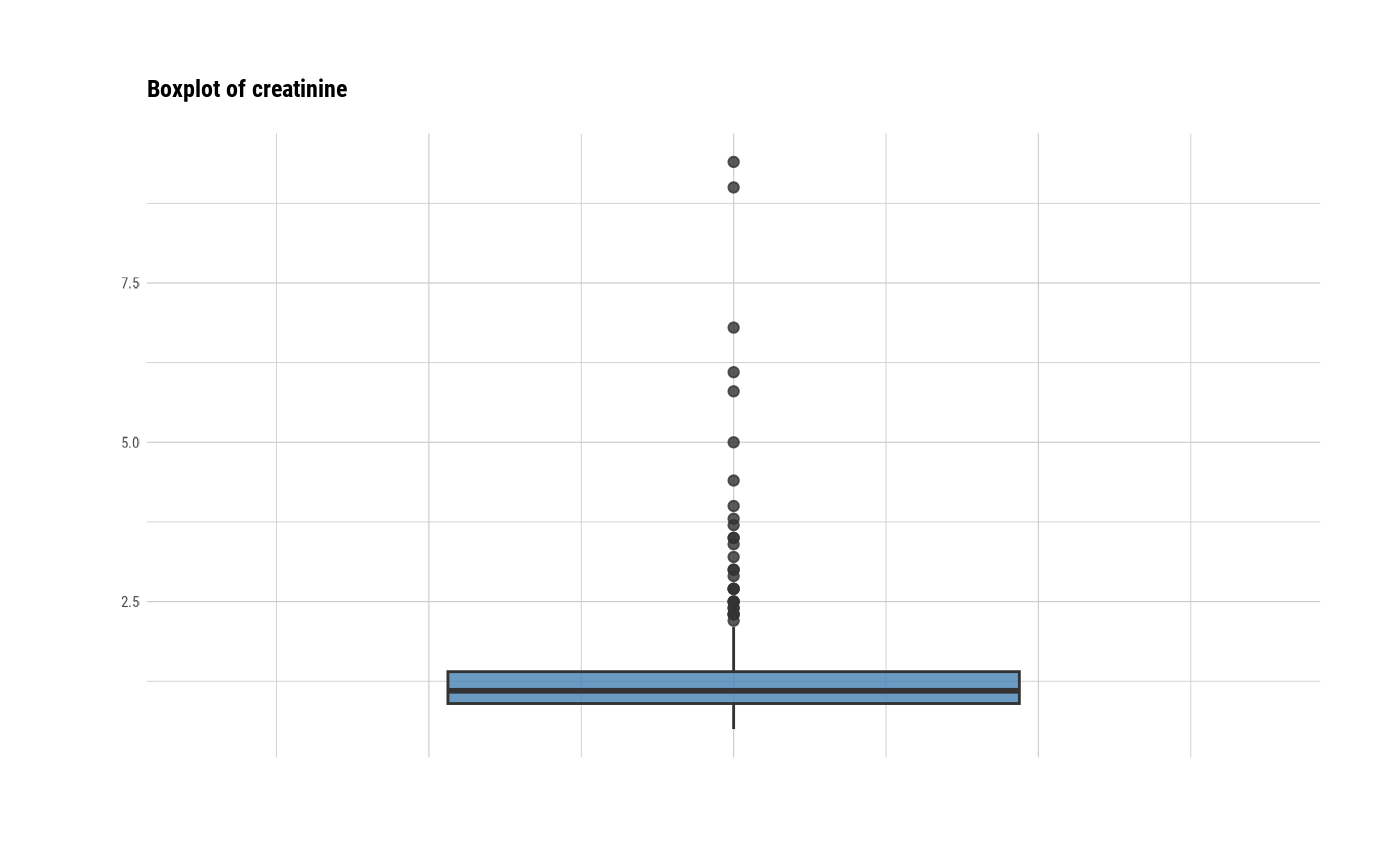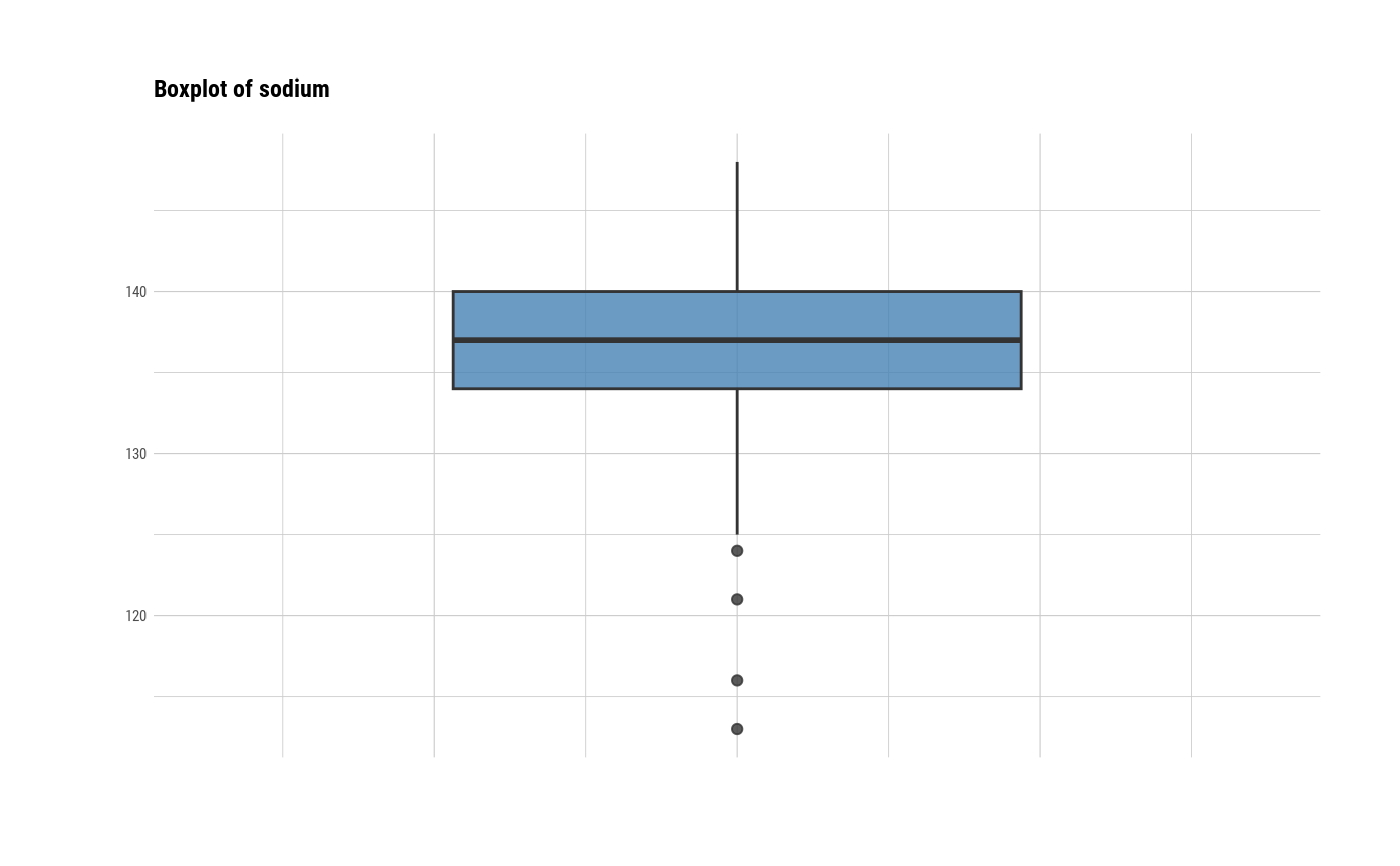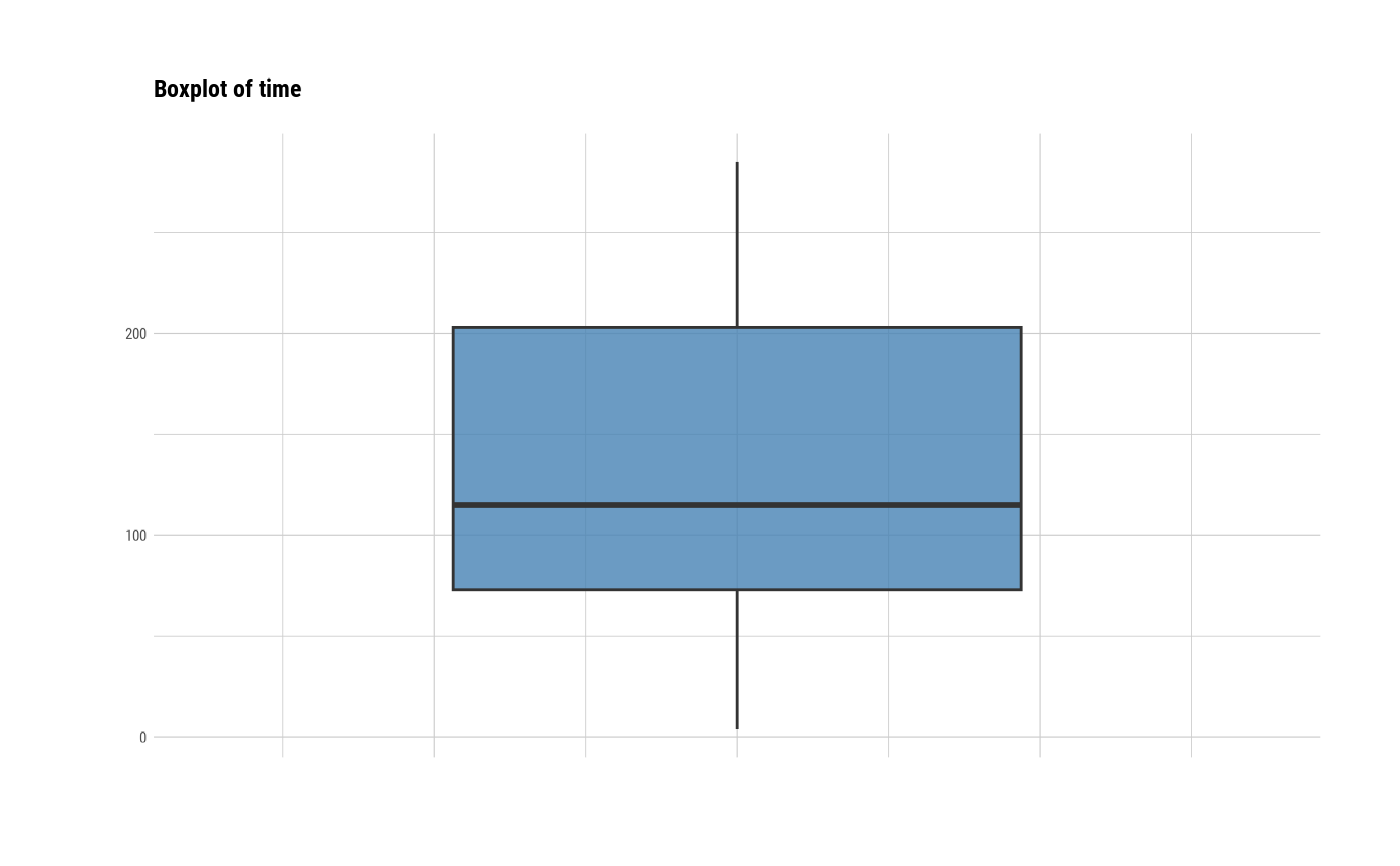# individual histogram by variables
plot(all_var, indiv = TRUE, "hist")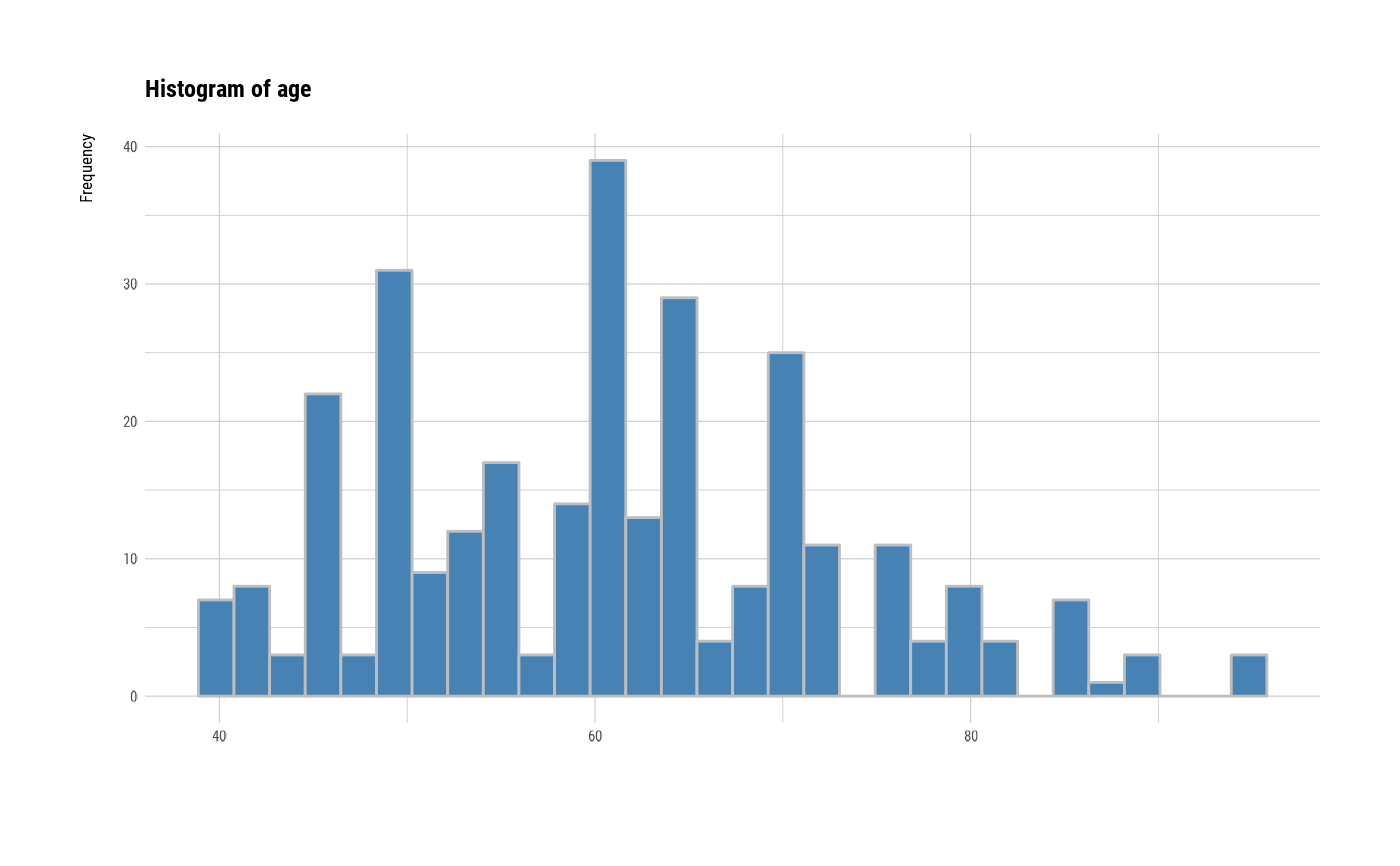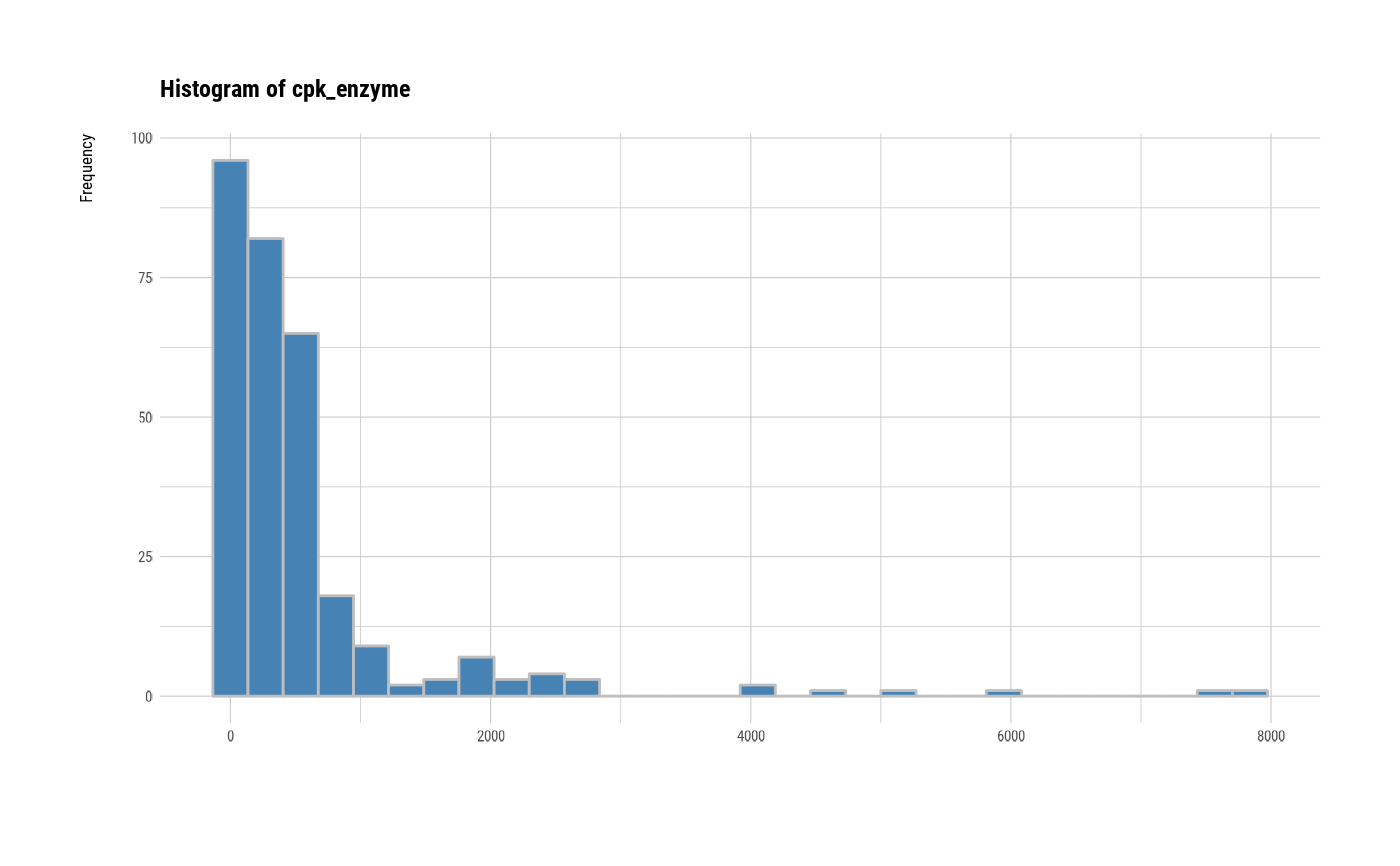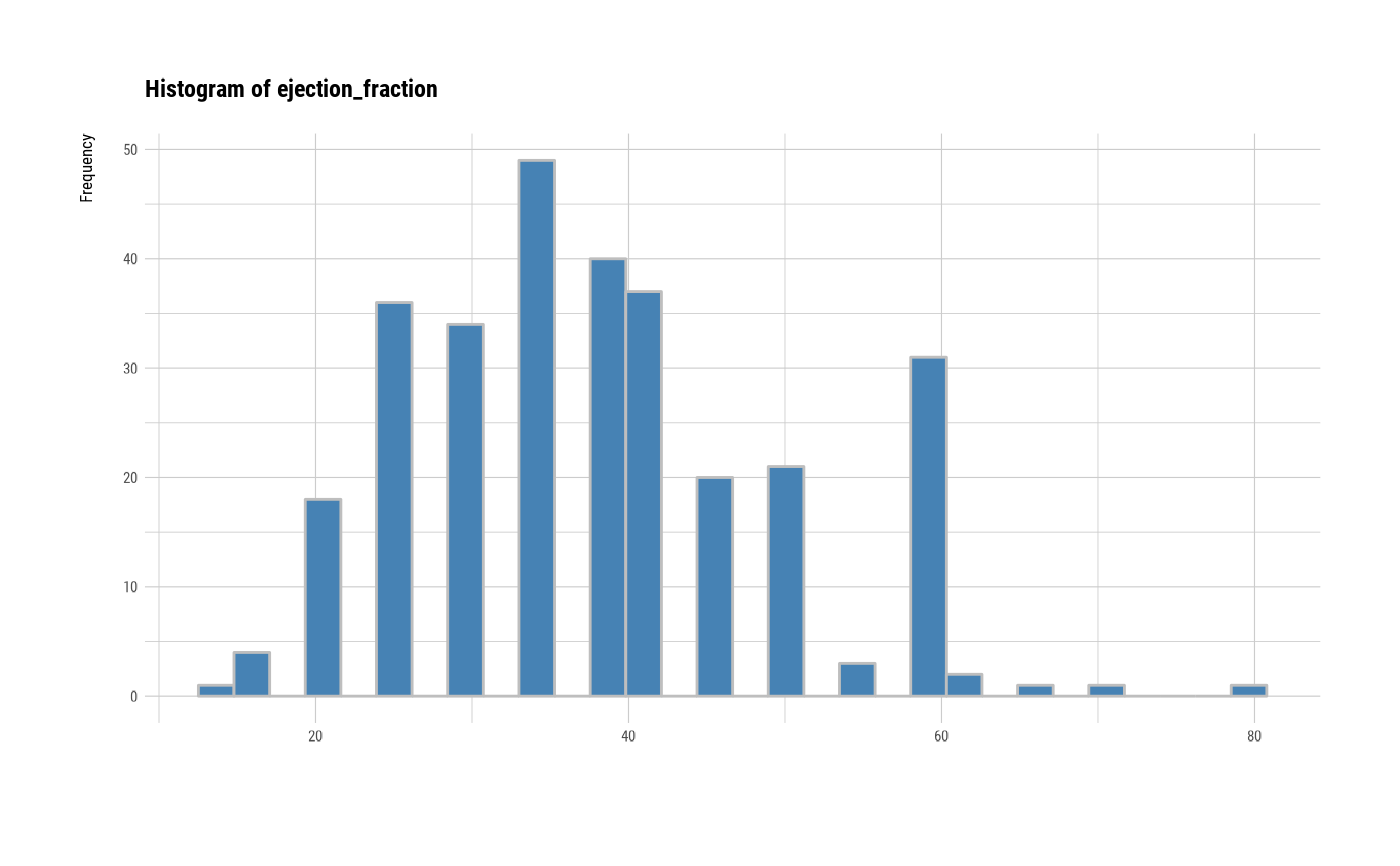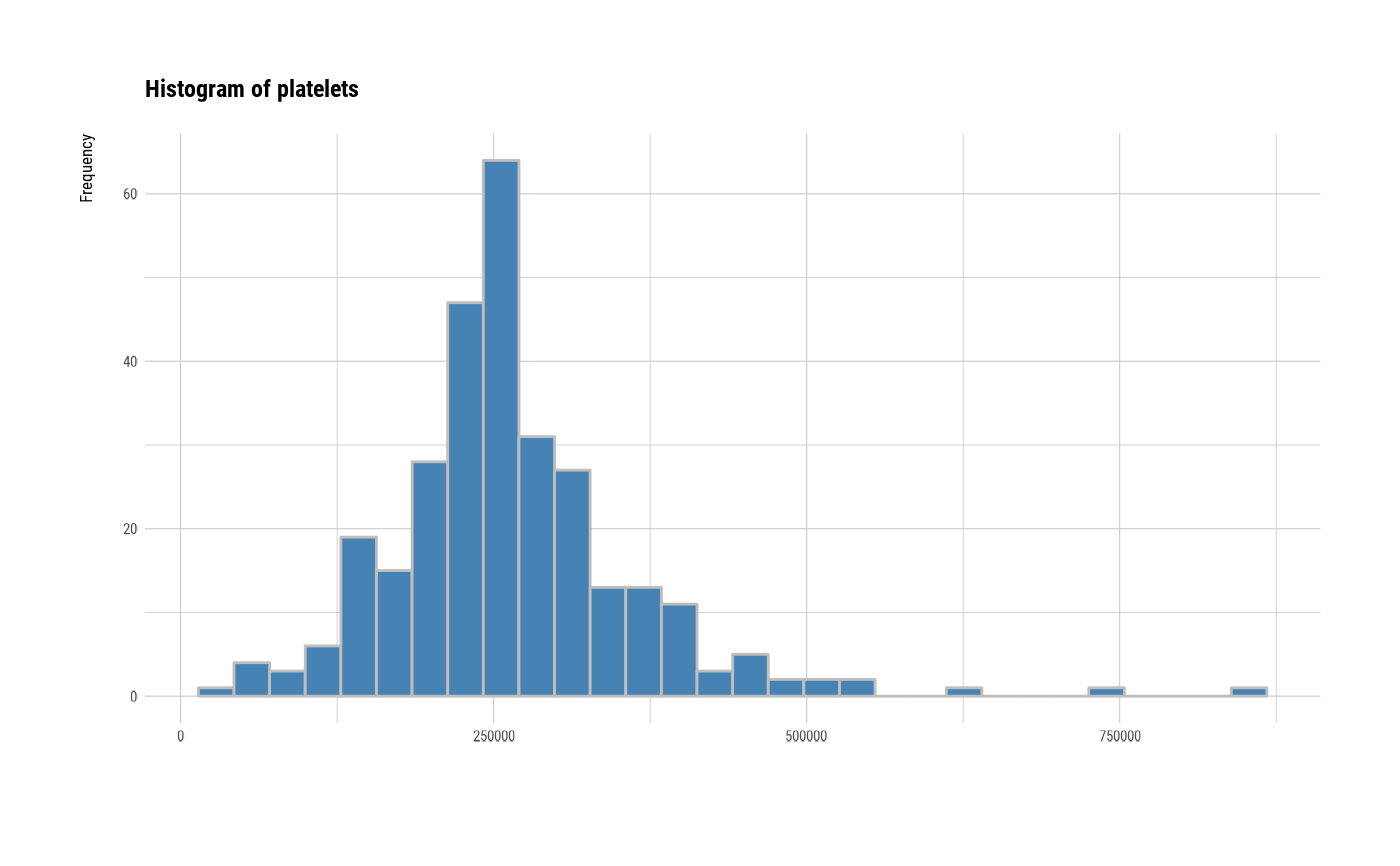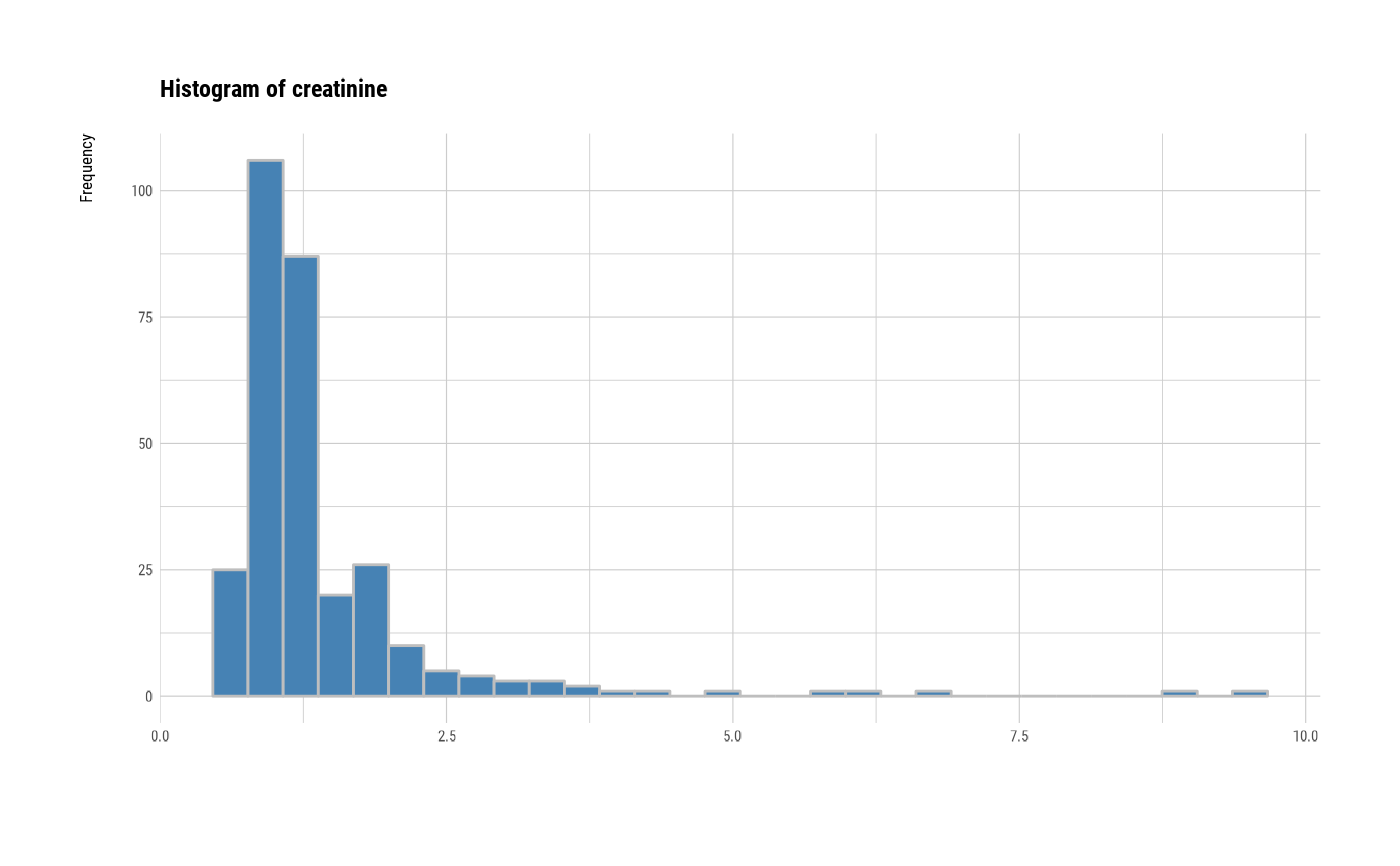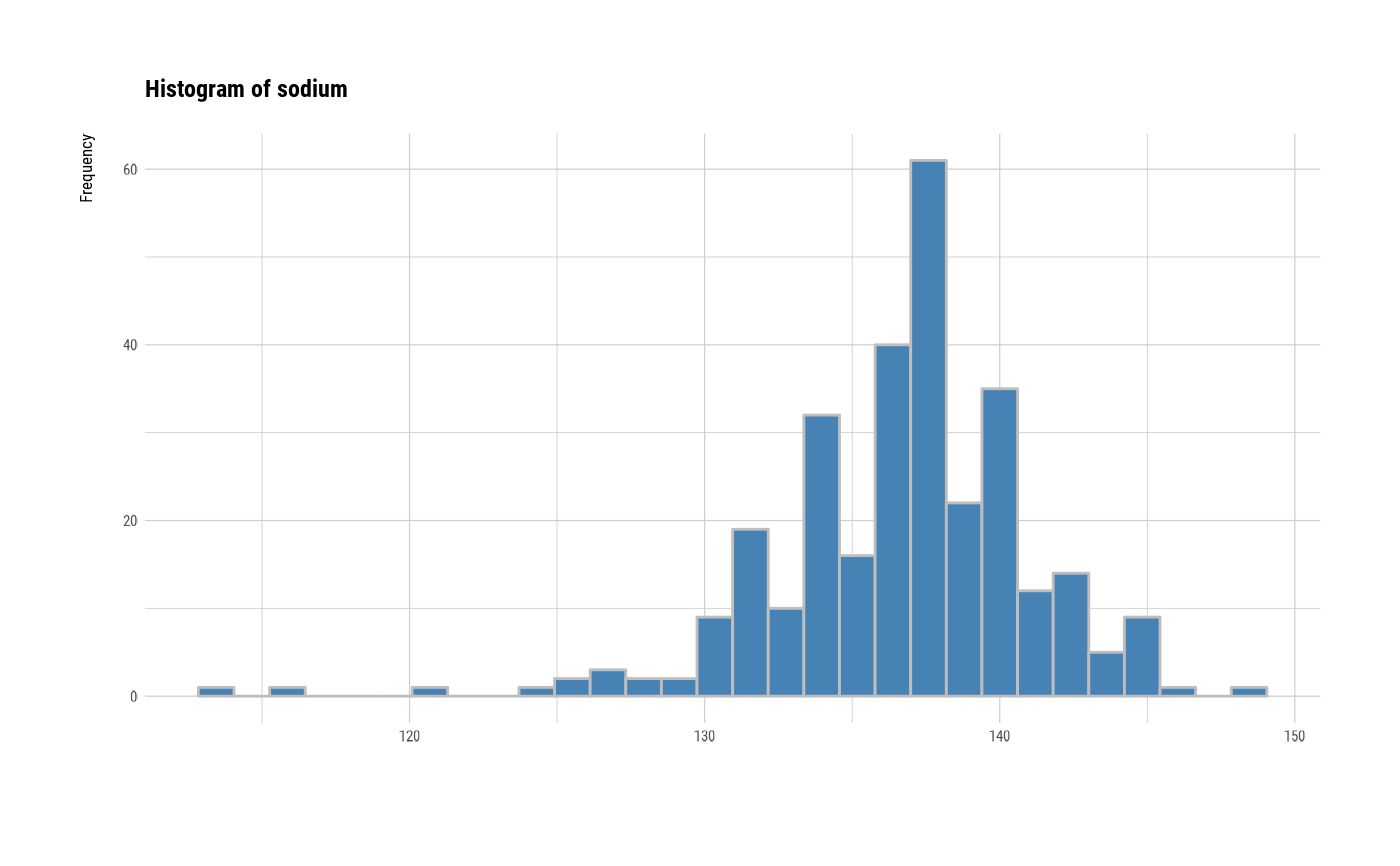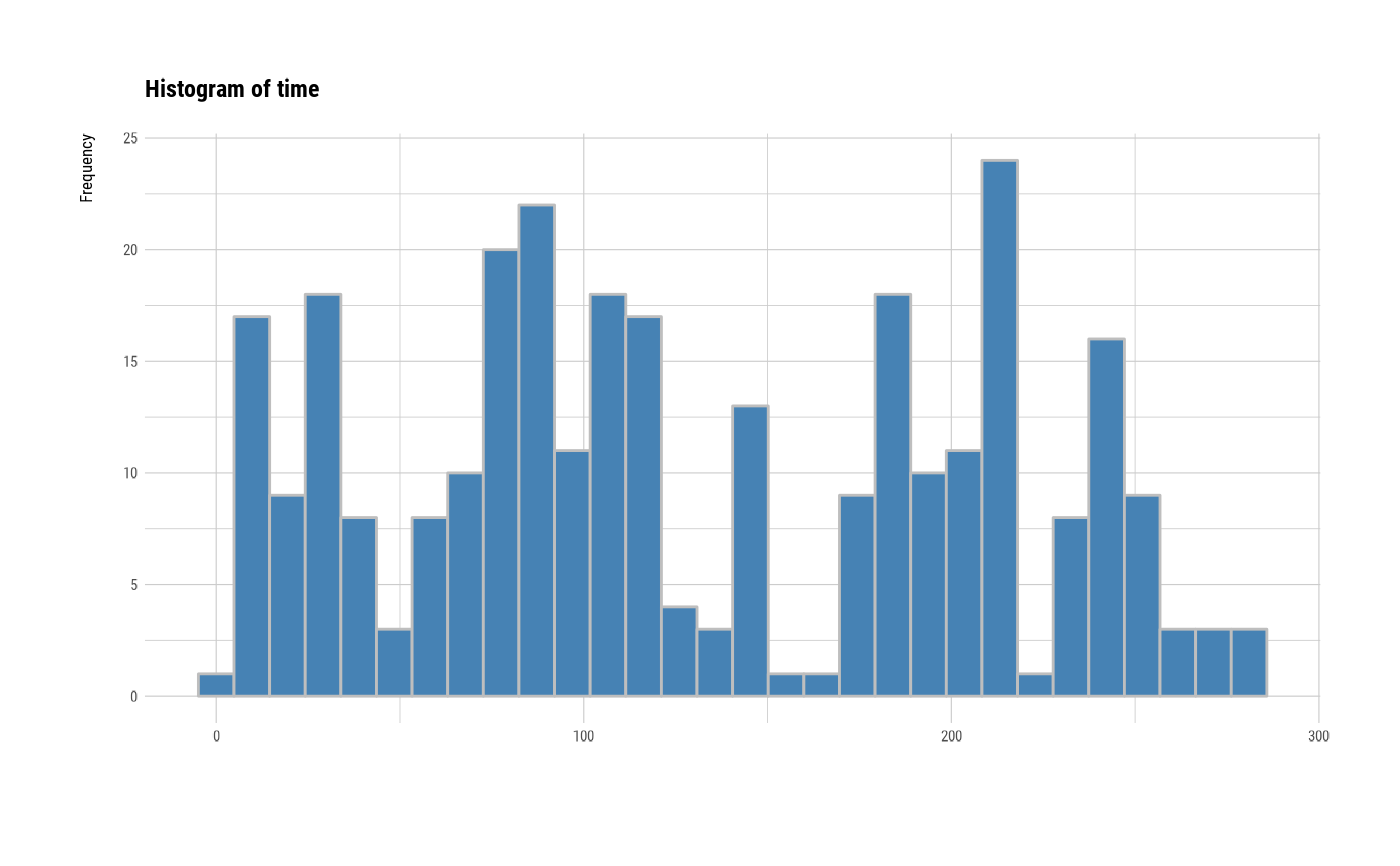# individual histogram by robust standardized variable
plot(all_var, indiv = TRUE, "hist", stand = "robust")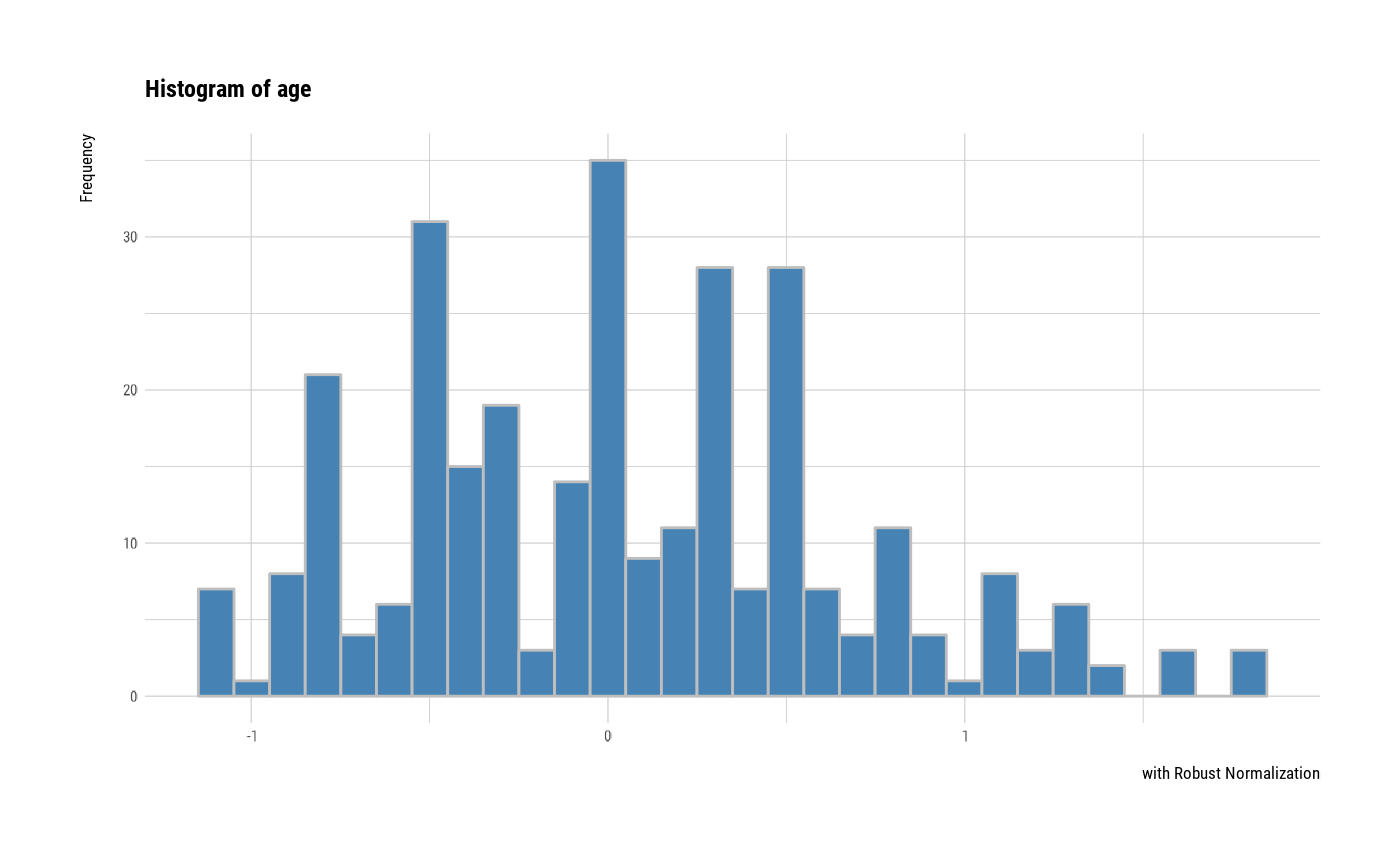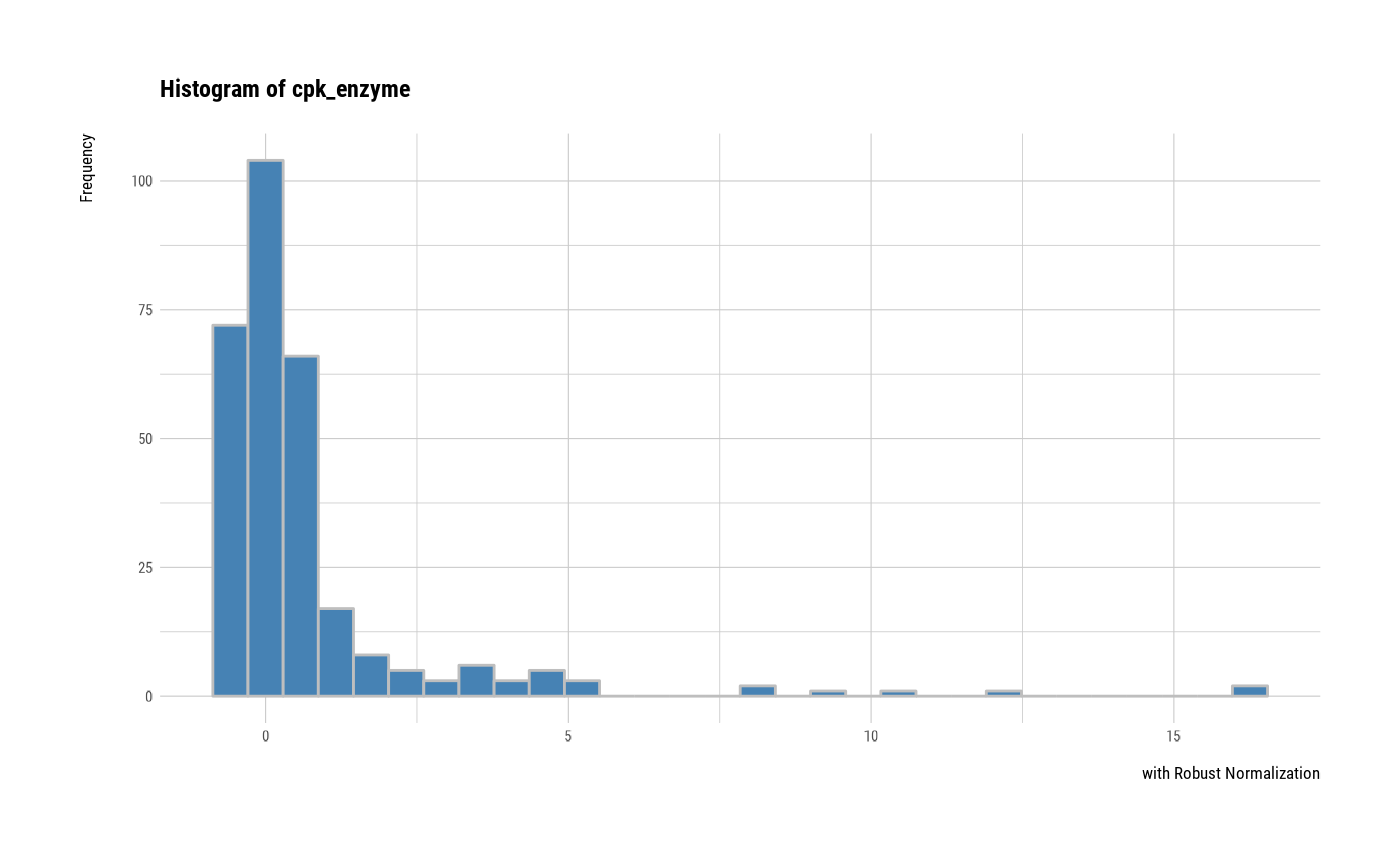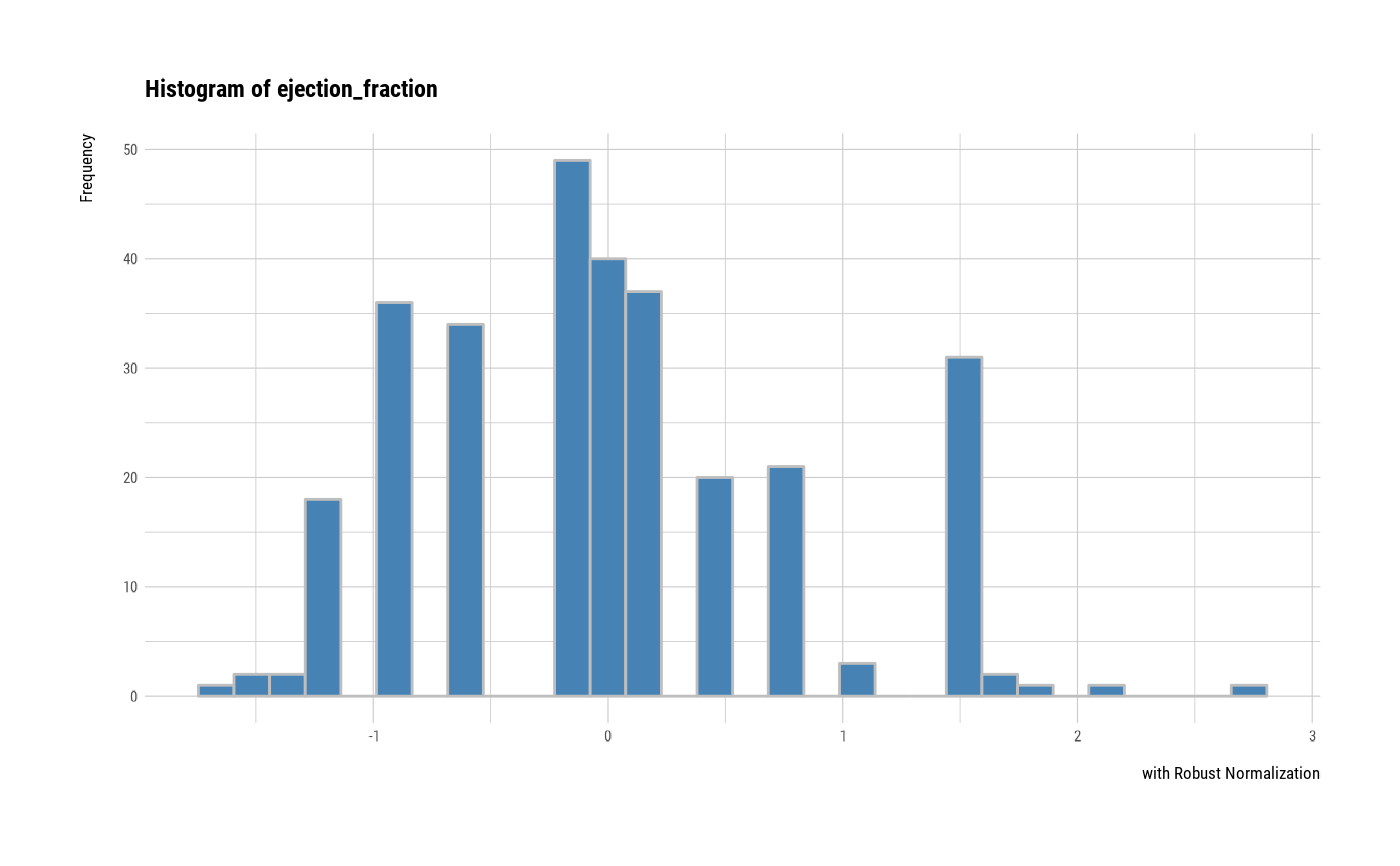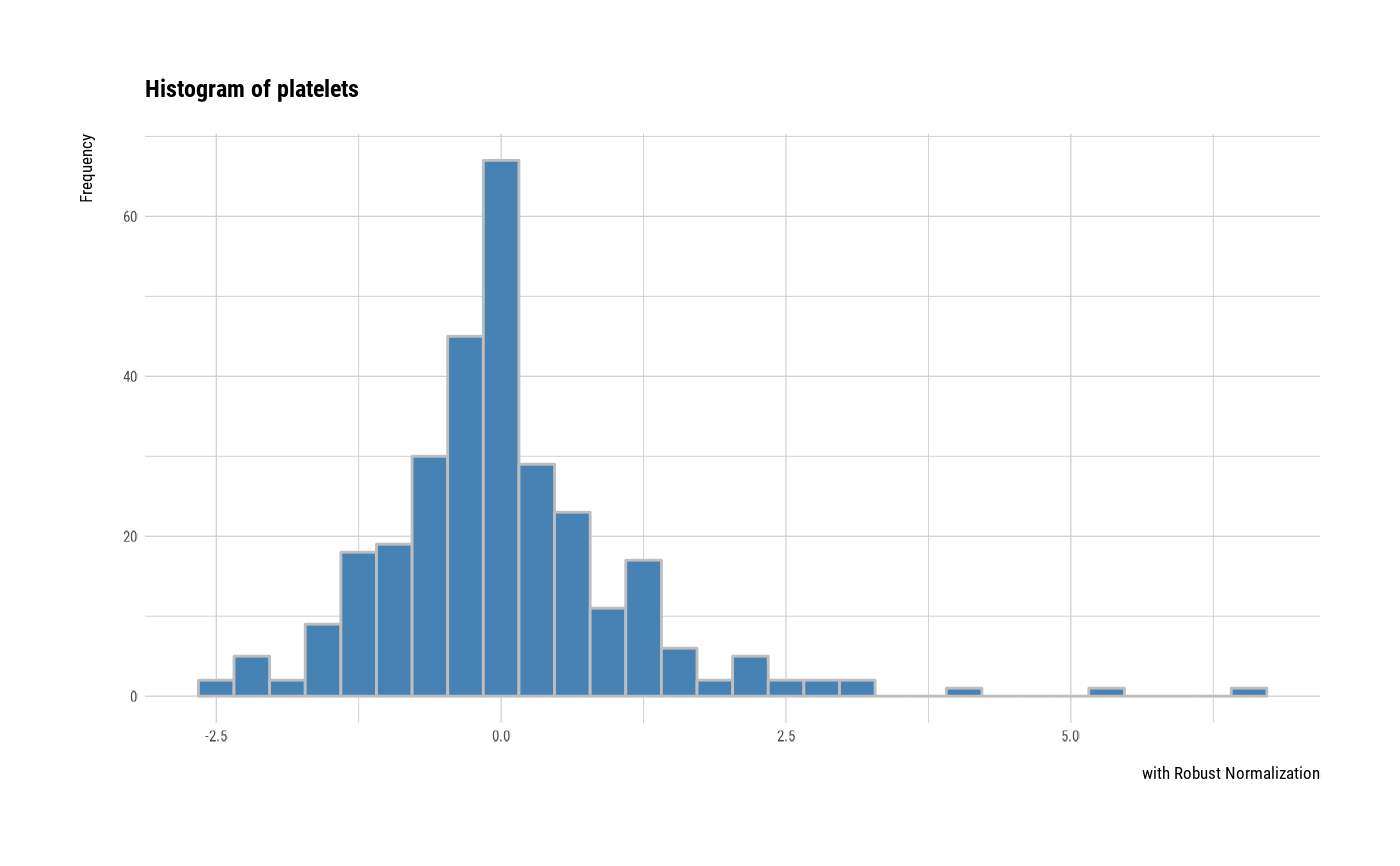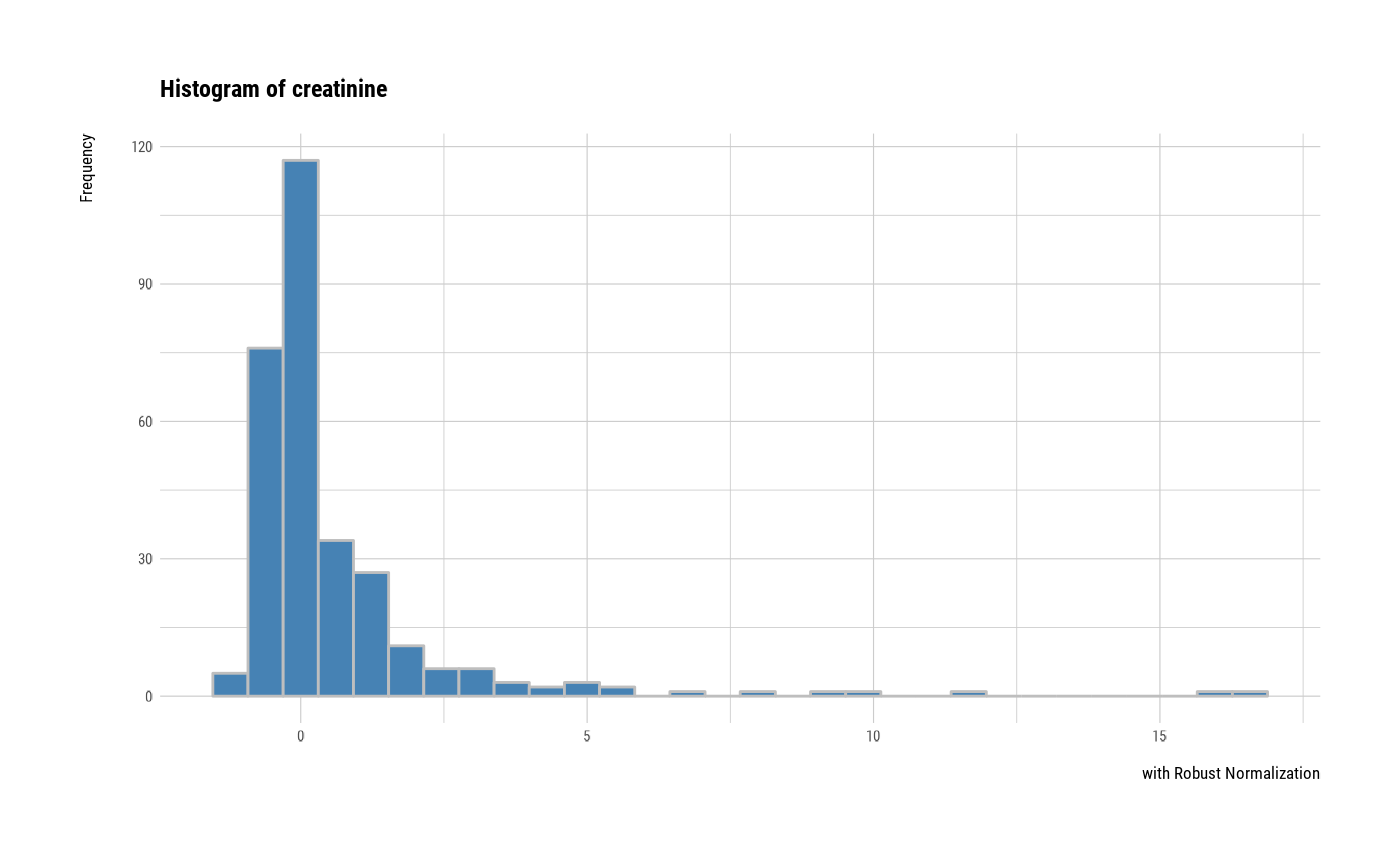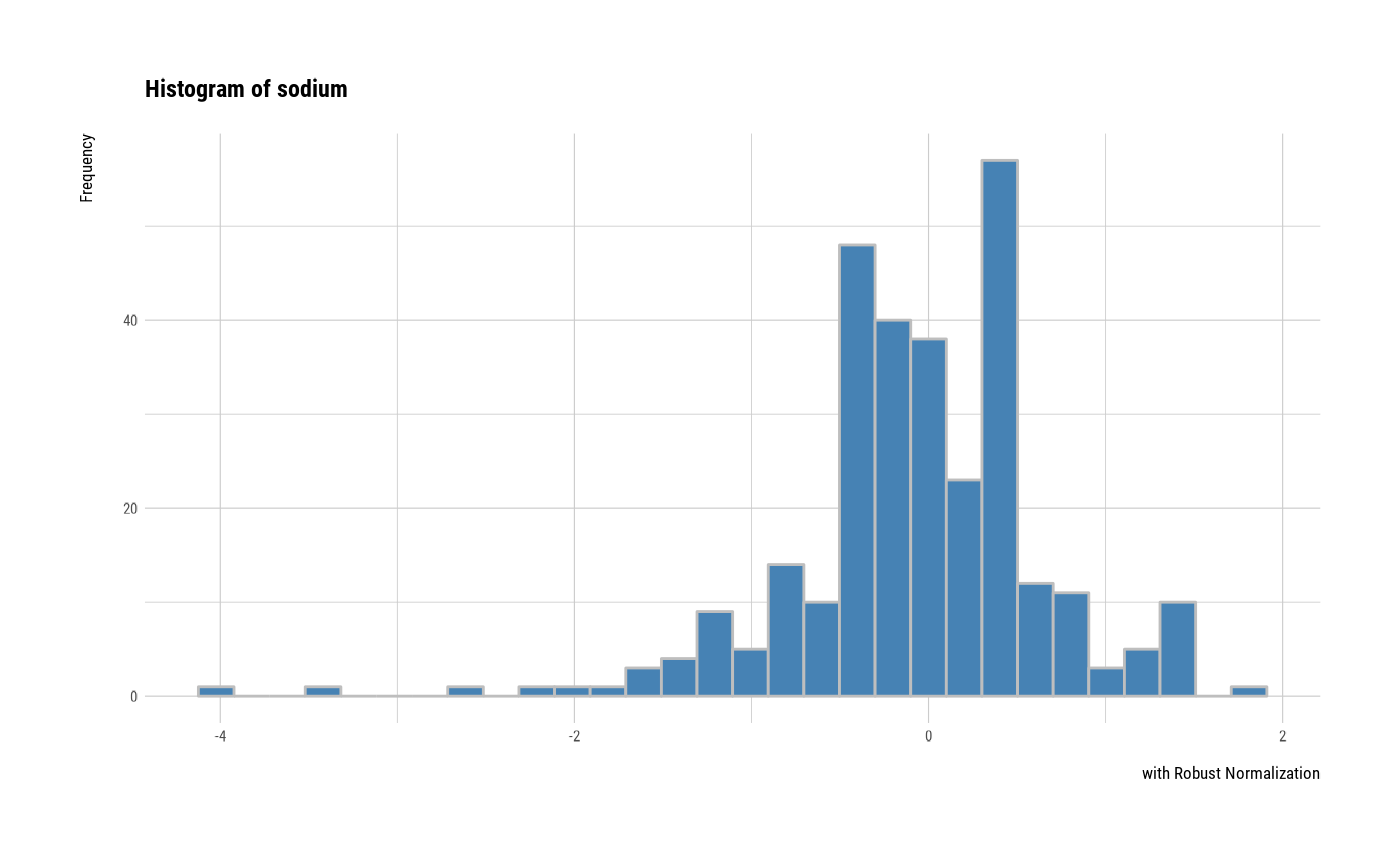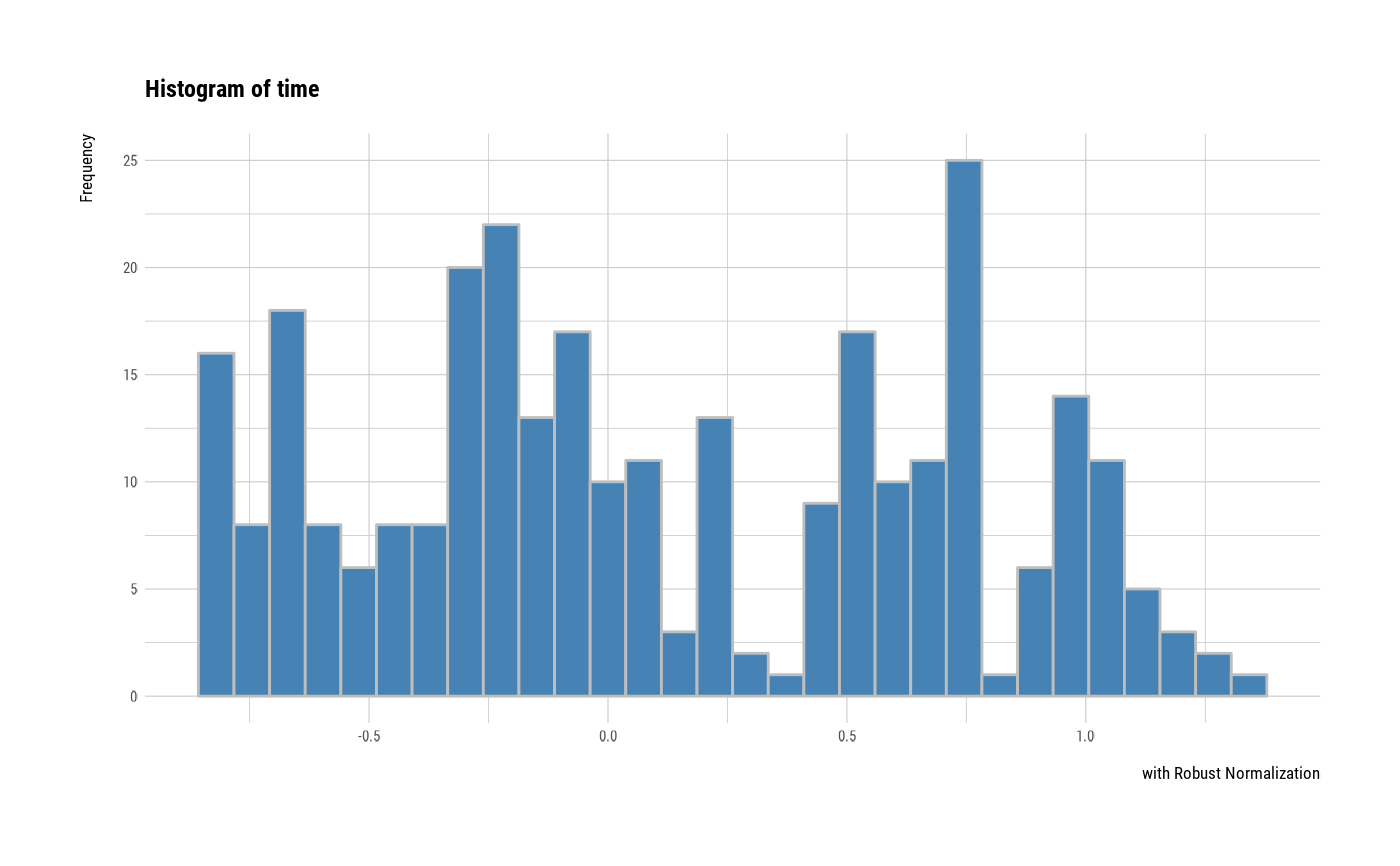# plot all variables by prompt
plot(all_var, indiv = TRUE, "hist", prompt = TRUE)# }